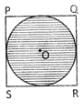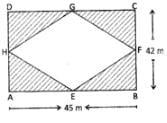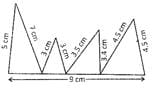Test: Area And Perimeter - 2

# Test: Area And Perimeter - 2

Test Description

## 10 Questions MCQ Test Mathematics for Class 4: NCERT | Test: Area And Perimeter - 2

Test: Area And Perimeter - 2 for Class 4 2022 is part of Mathematics for Class 4: NCERT preparation. The Test: Area And Perimeter - 2 questions and answers have been prepared according to the Class 4 exam syllabus.The Test: Area And Perimeter - 2 MCQs are made for Class 4 2022 Exam. Find important definitions, questions, notes, meanings, examples, exercises, MCQs and online tests for Test: Area And Perimeter - 2 below.
Solutions of Test: Area And Perimeter - 2 questions in English are available as part of our Mathematics for Class 4: NCERT for Class 4 & Test: Area And Perimeter - 2 solutions in Hindi for Mathematics for Class 4: NCERT course. Download more important topics, notes, lectures and mock test series for Class 4 Exam by signing up for free. Attempt Test: Area And Perimeter - 2 | 10 questions in 20 minutes | Mock test for Class 4 preparation | Free important questions MCQ to study Mathematics for Class 4: NCERT for Class 4 Exam | Download free PDF with solutions
 1 Crore+ students have signed up on EduRev. Have you?
Test: Area And Perimeter - 2 - Question 1

### PQRS is a square in which PQ = 2.8 m. find the area of unshaded region.Test: Area And Perimeter - 2 - Question 2

### Length of one of the equal sides of an isosceles triangle is 9 cm. If length of unequal side is 3 cm less than the sum of length of equal sides, find the perimetre of the triangle.

Test: Area And Perimeter - 2 - Question 3

### Perimetre of a square is 20 cm, find the area of the square.

Test: Area And Perimeter - 2 - Question 4

ABCD is a rectangle in which AB = 45m and BC = 42m and E, F, G and H are the mid point of the sides AB, BC, CD, and DA. Find the cost of lying tiles in the shaded part, if cost of lying tiles is Rs. 5 per m2Test: Area And Perimeter - 2 - Question 5

Find the perimetre of the following figure,Test: Area And Perimeter - 2 - Question 6

Find the area of the circle whose perimetre is 1.32 cm.

Test: Area And Perimeter - 2 - Question 7

Length of one side of an equilateral triangle is 126 cm. Find the perimetre of the triangle.

Test: Area And Perimeter - 2 - Question 8

Length of a rectangle is twice of the breadth. If area of the rectangle is 18 cm2, find the perimetre of the rectangle.

Test: Area And Perimeter - 2 - Question 9

Height and base of a triangle are 20 cm and 24 cm respectively. Find the area of the triangle.

Test: Area And Perimeter - 2 - Question 10

Diametre of a circle is 0.56 cm. Find the perimeter of the circle.

## Mathematics for Class 4: NCERT

24 videos|44 docs|32 tests
 Use Code STAYHOME200 and get INR 200 additional OFF Use Coupon Code
Information about Test: Area And Perimeter - 2 Page
In this test you can find the Exam questions for Test: Area And Perimeter - 2 solved & explained in the simplest way possible. Besides giving Questions and answers for Test: Area And Perimeter - 2, EduRev gives you an ample number of Online tests for practice

## Mathematics for Class 4: NCERT

24 videos|44 docs|32 tests